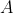# Basis maths-linear algebra-Q8

+1 vote

A pivot position in a matrixis a location in any row ofthat corresponds to a leading 1 in the reduced echelon form of.  A pivot column is a column ofthat contains a pivot position Or equivalently, A pivot position in a matrixis a location in any row ofthat corresponds to a leading entry in a row (left most non-zero entry) in any echelon form of.  A pivot column is a column of A that contains a pivot position.

Suppose the coefficient matrix of a linear system (L) of three equations in three unknowns(variables) has a pivot position in every column.

Which of the following is True?

(A). System L is consistent and has unique solution

(B). System L is consistent and has infinitely many solutions

(C). System L is inconsistent

(D). System is consistent but  Information is not sufficient to say whether there is a unique solution or infinitely many solutions.asked May 15
reshown May 16answered Jun 26 by (112,390 points)